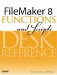# Factorial()

Category: Number

 Syntax: Factorial (number {; numberOfFactors })

Parameters:

numberAny expression that resolves to a positive integer.

numberOfFactorsAny expression that resolves to a positive integer that represents how many factors to include in the multiplication.

Parameters in curly braces { } are optional.

Data type returned: Number

Description:

Returns the factorial of number, stopping either at 1 or stopping at the optional numberOfFactors. The factorial of a number n is defined as nx(n1)x(n2)x(n3)...x1. Factorials are useful in statistics and combinatorics. In mathematics, factorials are usually represented by an exclamation mark. 4! = Factorial (4) = 4x3x2x1 = 24.

One application of factorials is to determine how many unique ways a set of objects can be ordered. For instance, a set of three objects {A, B, C} can be ordered 3! = 6 ways: {ABC, ACB, BAC, BCA, CAB, CBA}.

Examples:

Function

Results

Factorial ( 3 )

Returns 6, which = 3x2x1.

Factorial ( 10; 3 )

Returns 720, which = 10x9x8.FileMaker 8 Functions and Scripts Desk Reference
ISBN: 0789735113
EAN: 2147483647
Year: 2004
Pages: 352